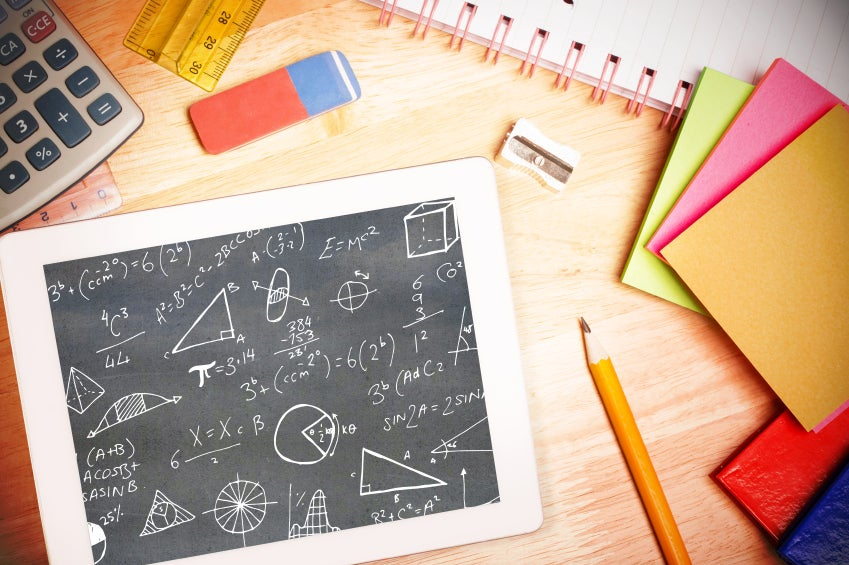# Math Project Ideas for the Ninth GradeThe Editorial Team January 1, 2013

Article continues here

Ninth grade math class introduces abstract concepts into the classroom. Quadratic formula, linear equations and more advanced geometry concepts are common examples, and many students ask, “When do we ever need to use this?!”

Ninth grade math projects can make math interesting, while also showing the real-world value of certain concepts. For students who are struggling to get excited about math, projects like these can make the subject exciting and relevant.

## Probability

Probability can be conceptual for students, so a project-based model for teaching how it works in the real world can help to solidify the concepts. Create a scenario where your students are working for a mall that is adding stores geared toward teenagers, and have them work as “consultants” for one of the stores.

Once the scenario is established, the students will interview the school to find out what trends and styles would sell best in their stores, then use their math skills and the data gathered to determine the probability of any given group of students, such as girls of a certain age range, wearing a specific style. The information can then be presented to the class, mirroring how an actual consultant would use technology and data to find a solution for a client.

## Bridge Building

Building bridges incorporates geometry, algebra and physics in a real-world application. Students can work in groups to build bridges out of a variety of class-friendly materials, like manila folders or flexible sticks. Teachers can even consider making a bridge building competition out of the assignment.

Teachers provide instruction on solid bridge-building shapes and techniques, and then students work together to create a bridge that is both lightweight and sturdy. Through trial and error, they will learn for themselves about what geometric shapes work best for trusses and supports.

## Candy Hunt

Once students know the slope-intercept form of a linear equation, they can use candy to write and solve systems in a hands-on way. Students are given graph paper, candy and a ruler and divided into groups of two. One group will choose a point on the graph to be the spot where the candy is “hidden.” Using that coordinate value, the students will write five separate equations. Three of them will have solutions that create a small area around the hidden candy’s point. The other two will have a solution that shows the exact location of the candy.

Once each group has their equations written, they exchange equations with another group. The groups then solve each other’s equations, graphing them on graph paper. If done correctly, they will be able to find the location of the hidden candy, at which point they get to eat the other group’s candy. If a group does not successfully find the location, the group that wrote the equations gets to keep the candy. If the group that wrote the equations made an error in writing the equations, then they must surrender their candy.

Ninth grade math projects that are hands-on in nature create excitement in the classroom. These projects get students out of the textbooks and interacting with numbers in a more tangible way. Students who normally do not care for math may find themselves excited about the subject. Projects like these work well alongside traditional math instruction to create an engaging, exciting math class.

Share